4906.0 - Personal Safety, Australia, 2016
Latest ISSUE Released at 11:30 AM (CANBERRA TIME) 08/11/2017
 Page tools: .mffeedback,#pjs { display :none; } Enable Javascript to Print PagesPrint AllEmail NotificationRSSSearch this Product Explanatory Notes Glossary Abbreviations Technical Note TECHNICAL NOTE RELIABILITY OF ESTIMATES 1 The estimates in the 2016 PSS publication are based on information obtained from a sample of the Australian population. Although care has been taken to ensure that the results of the survey are as accurate as possible, there are certain factors which can affect the reliability of the results to some extent and for which no adequate adjustments can be made. 2 One such factor is known as sampling error. The key measures used to assess the impact of sampling error on the 2016 PSS estimates in this publication are described below. Such calculations were undertaken in this publication and should be kept in mind when interpreting the results of this survey. 3 Other factors are collectively referred to as non-sampling errors. For more details on sampling error as well as details on non-sampling errors, refer to the Data Quality and Technical Notes page in the Personal Safety Survey, Australia: User Guide, 2016 (cat. no. 4906.0.55.003). Sampling error 4 As the 2016 PSS data was obtained from a sample of the Australian population, the impact of sampling error on estimates was closely reviewed. Sampling error (or sampling variability) is used to describe the circumstance where survey estimates differ from those that would have been produced had all persons been included in the survey. The magnitude of the sampling error associated with a sample estimate depends on the following factors: Sample design - the final design attempted to make key survey results as representative as possible within cost and operational constraints. Sample size - the larger the sample on which the estimate is based, the smaller the associated sampling error. Population variability - the extent to which people differ on the particular characteristic being measured. This is referred to as the population variability for that characteristic. The smaller the population variability of a particular characteristic, the more likely it is that the population will be well represented by the sample, and, therefore the smaller sampling error. Conversely, the more variable the characteristic, the greater the sampling error. Calculation of standard error 5 One measure of the likely difference in estimates is given by the Standard Error (SE), which indicates the extent to which an estimate might have varied because only a sample of dwellings was included. There are about two chances in three (67%) that the sample estimate will differ by less than one SE from the figure that would have been obtained if all dwellings had been included, and about 19 chances in 20 that the difference will be less than two SEs.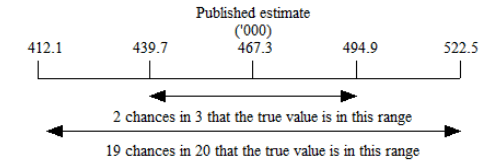6 For estimates of population sizes, the size of the SE generally increases with the level of the estimate, so that the larger the estimate, the larger the SE. However, the larger the sampling estimate the smaller the SE becomes in percentage terms. Thus, larger sample estimates will be relatively more reliable than smaller estimates. SE can be calculated using the estimates (counts or percentages) and the corresponding Relative Standard Error (RSE). For example, in this publication the estimated males aged 18 years and over who experienced physical assault in the last 12 months was 309,400. The RSE corresponding to this estimate is 8.7%. The SE is calculated by: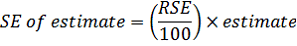= (8.7 / 100) * 309400 = 26,900 (rounded to the nearest 100) 7 The RSE is obtained by expressing the SE as a percentage of the estimate to which it related. The RSE is a useful measure in that it provides an immediate indication of the percentage errors likely to have occurred due to sampling, and thus avoids the need to refer also to the size of the estimate.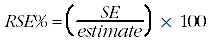8 Estimates with RSEs less than 25% are considered sufficiently reliable for most purposes. However, estimates with RSEs of 25% or more are included in this publication of results and have been appropriately identified to use with caution. RSEs are presented in the tables of the publication for estimates ('000). Estimates with RSEs greater than 25% but less than or equal to 50% are annotated with an asterisk (*) to indicate they are subject to high SEs relative to the size of the estimate and should be used with caution. Estimates with RSEs of greater than 50%, annotated with a double asterisk (**), are considered too unreliable for most purposes. These estimates can be aggregated with other estimates to reduce the overall sampling error. Note that RSEs for proportion estimates (%) are not presented in the tables of this publication, but rather the Margin of Error (MoE) is presented (see section below). However RSEs can be produced from the TableBuilder or Detailed Microdata products or by request. Calculation of Margin of Error 9 Another useful measure is the Margin of Error (MoE), which describes the distance from the population value that the sample estimate is likely to be within, and is specified at a given level of confidence. Confidence levels typically used are 90%, 95% and 99%. For example, at the 95% confidence level, the MoE indicates that there are about 19 chances in 20 that the estimate will differ by less than the specified MoE from the population value (the figure obtained if all dwellings had been enumerated). The MoE at the 95% confidence level is expressed as 1.96 times the SE. 10 A confidence interval expresses the sampling error as a range in which the population value is expected to lie at a given level of confidence. The confidence interval can easily be constructed from the MoE of the same level of confidence, by taking the estimate plus or minus the MoE of the estimate. In other terms, the 95% confidence interval is the estimate +/- MoE i.e. the range from minus 1.96 times the SE to the estimate plus 1.96 times the SE. The 95% MoE can also be calculated from the RSE by the following, where y is the value of the estimate: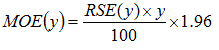11 Note due to rounding, the SE calculated from the RSE may be slightly different to the SE calculated from the MoE for the same estimate. The SE of estimate using MoEs is calculated by: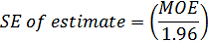12 Using the two formulas above, it was found that there are about 19 chances in 20 that the estimate of the proportion of females aged 18 years and over who experienced sexual harassment in the last 12 months (17.3%) is within +/- 1.1 percentage points from the population value. Similarly, there are about 19 chances in 20 that the proportion of females aged 18 years and over who experienced sexual harassment in the last 12 months is within the confidence interval of 16.2% to 18.4%. 13 In the tables in this publication, MoEs are presented for the proportion estimates (%). Proportion estimates are preceded by a hash (e.g. #10.2) if the corresponding MoE is greater than 10 percentage points. An estimate is also preceded by a hash if the MoE is large enough such that the corresponding confidence interval for this estimate would exceed the value of 0% and/or 100%; the natural limits of a proportion. The latter situation will occur if the MoE is greater than the estimate itself, or greater than 100 minus the estimate. Users should give the margin of error particular consideration when using this estimate. Note that MoEs for 1996 proportion estimates in the tables for this publication were calculated using the RSEs presented in the RSE tables found in the Women’s Safety Survey, 1996 (cat. no. 4128.0). Standard error of a difference 14 The difference between two survey estimates is itself an estimate and is therefore subject to sampling error or variability. The sampling error of the difference between the two estimates depends on their individual SEs and the level of statistical association (correlation) between the estimates. An approximate SE of the difference between two estimates (x-y) may be calculated by the following formula:15 For example, the number of females who have been stalked minus the number of males who have been stalked. While this formula will only be exact for differences between separate sub-populations or uncorrelated characteristics of sub-populations, it is expected to provide a reasonable approximation for most differences likely to be of interest in relation to this survey. Significance testing on differences between survey estimates 16 When comparing estimates between surveys or between populations within a survey, it is useful to determine whether apparent differences are 'real' differences between the corresponding population characteristics or simply the product of differences between the survey samples. One way to examine this is to determine whether the difference between the estimates is statistically significant. A statistical significance test for a comparison between estimates can be performed to determine whether it is likely that there is a difference between the corresponding population characteristics. The standard error of the difference between two corresponding estimates (x and y) can be calculated using the formula shown above in the Standard error of a difference section. This standard error is then used to calculate the test statistic: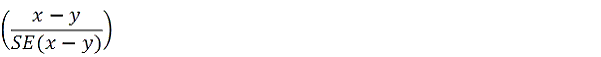17 If the value of this test statistic is greater than 1.96 then there is good evidence, with a 95% level of confidence, of a statistically significant difference in the two populations with respect to that characteristic. Otherwise, it cannot be stated with confidence (at the 95% confidence level) that there is a real difference between the populations. 18 Data presented in the commentary chapters of this publication have been significance tested to assess whether or not there is a difference (for example, between men and women) or change (for example between 2012 and 2016). When undertaking additional analysis of data presented in the tables, significance testing is recommended. Example of estimates where there was a statistically significant difference 19 An estimated 5.4% of all men aged 18 years or over and 3.5% of all women aged 18 years or over had experienced physical violence during the 12 months prior to the survey. The estimate of 5.4% of men who had experienced physical violence in the 12 months prior to the survey has an RSE of 7.0%. There are 19 chances out of 20 that an estimate of between 4.7% and 6.1% (or +/- 0.7% MoE) of men would have been obtained if all dwellings had been included in the survey. The estimate of 3.5% of women who had experienced physical violence in the 12 months prior to the survey has an RSE of 5.9%. There are 19 chances out of 20 that an estimate of between 3.1% and 3.9% (or +/- 0.4% MoE) women would have been obtained if all dwellings had been included in the survey. The value of this test statistic, (at 4.62 using the formula shown in the significance testing section above), is greater than 1.96. This showed that there was evidence, with a 95% level of confidence, of a statistically significant difference in the two estimates. By calculating the confidence interval for the proportion of men and women who experienced physical violence in the 12 months prior to the survey, it can be seen that the confidence intervals for estimates for men and women do not overlap (where the confidence intervals do not overlap, there is always a statistically significant difference). Therefore there is evidence to suggest that men were more likely than women to have experienced physical violence in the 12 months prior to the survey. 20 For information on detailed reliability of estimates, refer to the Data Quality and Technical Notes page in the Personal Safety Survey, Australia: User Guide, 2016 (cat. no. 4906.0.55.003). Document Selection These documents will be presented in a new window.